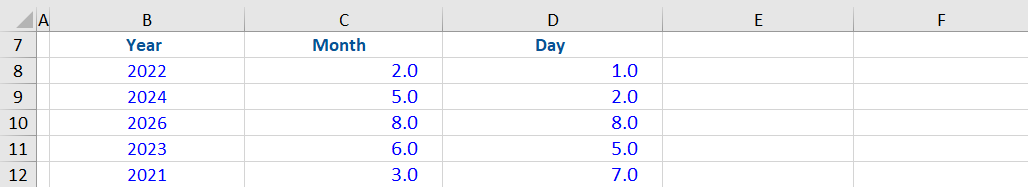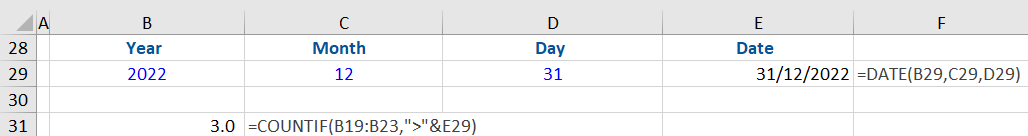## What is the DATE Function?

The DATE function is an Excel function that combines three separate values (year, month, and day) to form a date. When used along with other Excel functions, it can be used to perform a wide range of tasks related to dates, including returning specified dates. The function is also useful when dates are input to other functions such as SUMIF or COUNTIF.

## Key Learning Points

• The DATE function is a date/time function in Excel that combines three separate values to form a date
• The function, when combined with other Excel functions, has various applications in financial modeling
• The function is also useful when dates serve as an input to other functions, including SUMIF and COUNTIF
• The function has three required arguments: Year, Month, and Day
• #NUM! error is caused if the year argument is <0 or >=10000
• #VALUE! error is caused if non-numeric arguments are entered
• Excel displays dates as serial numbers as a default option. To view the results of this function as dates, you need to change the cell format to ‘Date’

## Syntax

The syntax for the DATE function is shown as:

Date(year, month, day)

The syntax includes the following required arguments:

• Year represents years whose value can range from a single digit (e.g., 2) to four digits (e.g., 1902). Microsoft Excel for Windows uses the 1900 date system as a default. So, if you enter a single digit ‘2’ in the year field, Excel will assume the year to be 1902. It is better to enter 4-digit years to avoid confusion.
• Month represents months of a year whose value can range from 1 to 12, depending on the month (starting from January). For example, if we enter 2 as an argument, the function will return the month of February.

We can also enter a negative integer as Month. For example, if we enter DATE (2025,-2,2) as arguments, the function will subtract 2 months from the first month of 2025.

• Day is the day of the month whose value can range from 1 to 31, representing the number of days in a month.

Similar to month, day can also be negative. Example, if we enter (2024,10,-2) as the day, it will subtract 2 days from the 1st day of October and show the date as September 28th, 2024.

## Important Points About the DATE Function

• If the year argument is less than 0 or greater than/equal to 10000, it results in a #NUM! error
• If a non-numeric argument is entered, it leads to a #VALUE! error
• By default, Excel stores dates as sequential numbers, starting from 01-01-1900 (which is 1 for Excel). The cell in which this function is entered needs to be set to the date format. This can be done by selecting the Date dropdown in the Number group (part of the Home tab). You can also change the format to date using the format cells option.

## Example 1:

Using the information given below, combine the year, month, and day to form a date. Download the free Excel file to test the Date Function yourself.The dates can be created in a single cell using the DATE function as shown below:## Example 2:

We have been asked to add 15 days to each of the DATE function results in the previous example.

To solve for the above, we need to add ‘+15’ to the formula.

*It is best practice to reference other cells in formulas instead of hardcoding. This allows you to update all affected formulas quickly rather than each one individually.The dates have changed to 15 days after the dates calculated using the original arguments.

We can also add years and months to any of the dates. For example, if we want to calculate the 10th anniversary of each of these dates, we will add 10 to the year argument while leaving the other arguments unchanged.

## Example 3:

From the information given above, calculate the number of dates after December 31st, 2022.

This is an example where the DATE function is used with other functions to get the desired result. Here, we will be using the COUNTIF function, which only counts cells that meet a specified condition.

As a first step, we assemble this date using the DATE function. This step gives us the flexibility to easily change the days, which the function can update automatically.Next, we use the COUNTIF function by selecting the date range and have asked it to count dates greater than the date in cell E29.There are 3 dates in the range after this date.